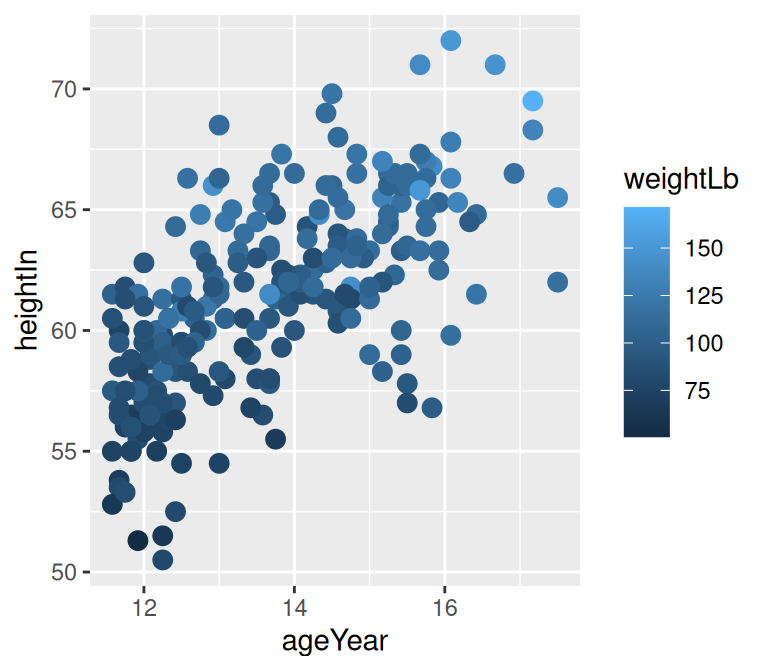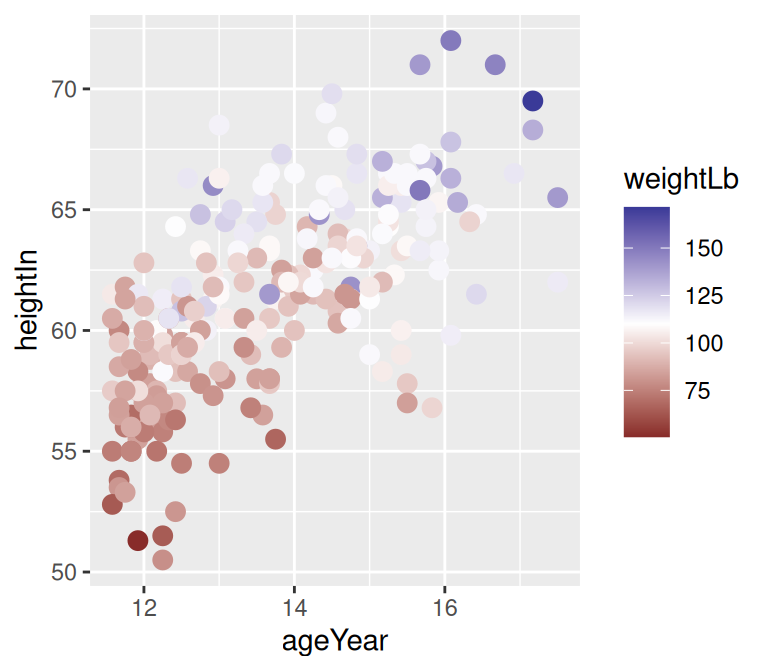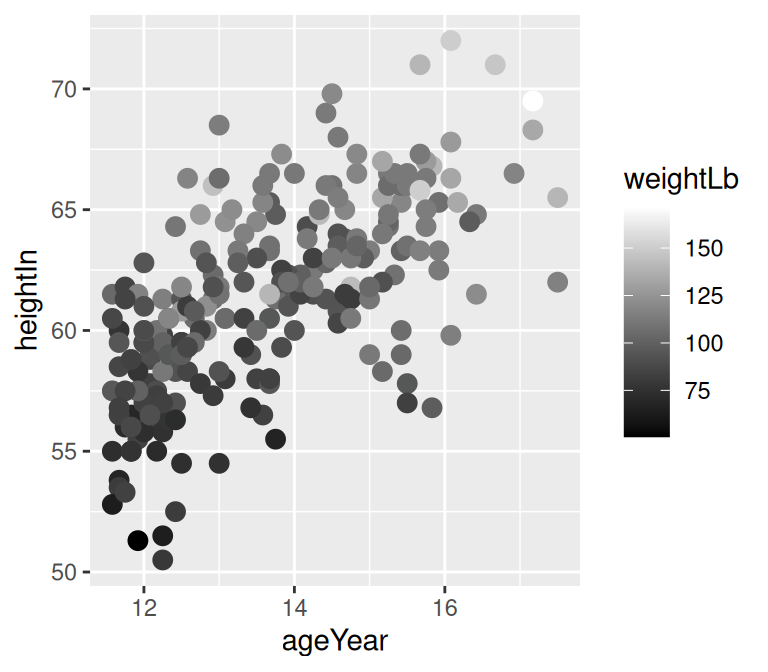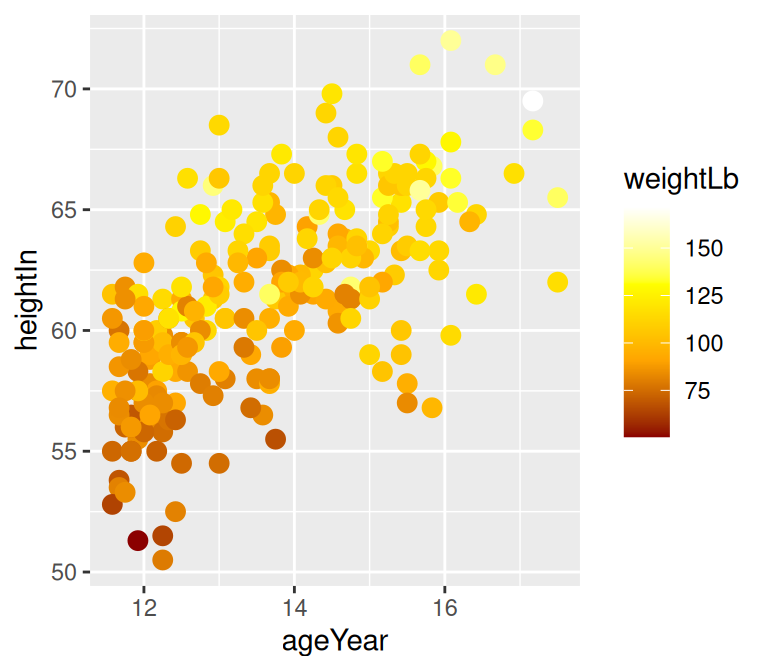## 12.6 Using a Manually Defined Palette for a Continuous Variable

### 12.6.1 Problem

You want to use different colors for a continuous variable.

### 12.6.2 Solution

In the example here, we’ll specify the colors for a continuous variable using various gradient scales (Figure 12.12). The colors can be named, or they can be specified with RGB values:

``````library(gcookbook)  # Load gcookbook for the heightweight data set

# Create the base plot
hw_plot <- ggplot(heightweight, aes(x = ageYear, y = heightIn, colour = weightLb)) +
geom_point(size = 3)

hw_plot

# A gradient with a white midpoint
library(scales)
hw_plot +
low = muted("red"),
mid = "white",
high = muted("blue"),
midpoint = 110
)

# With a gradient between two colors (black and white)
hw_plot +
scale_colour_gradient(low = "black", high = "white")

# A gradient of n colors
hw_plot +
scale_colour_gradientn(colours = c("darkred", "orange", "yellow", "white"))``````Figure 12.12: Clockwise from top left: default colors, two-color gradient (black and white) with `scale_colour_gradient()`, three-color gradient with midpoint with `scale_colour_gradient2()`, four-color gradient with `scale_colour_gradientn()`

For fill scales, use `scale_fill_xxx()` versions instead, where `xxx` is one of `gradient`, `gradient2`, or `gradientn`.

### 12.6.3 Discussion

Mapping continuous values to a color scale requires a continuously changing palette of colors. Table 12.2. lists the continuous color and fill scales.

Table 12.2: Continuous fill and color scales
Fill scale Color scale Description
`scale_fill_gradient()` `scale_colour_gradient()` Two-color gradient
`scale_fill_gradient2()` `scale_colour_gradient2()` Gradient with a middle color and two colors that diverge from it
`scale_fill_gradientn()` `scale_colour_gradientn()` Gradient with n colors, equally spaced
`scale_fill_viridis_c()` `scale_colour_viridis_c()` Viridis palettes

Notice that we used the `muted()` function in the examples. This is a function from the scales package that returns an RGB value that is a less-saturated version of the color chosen.